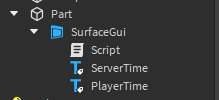# Displaying server and local time on a surface gui

I am trying to have the server uptime display on a surfacegui and then under it have the amount of time the local player has been in the server

Have a Script increment by 1 every second to track server time.
And have a local script increment by 1 every second to track player time.i think this is too easy to about

how would I do this? I am a really basic scripter

Sorry i was busy i’m finally able to write the code
here you go

1. Create a script and put it in the SurfaceGui and put this code in
``````function TimeFormat(seconds)
local hours = seconds / 3600
local left = hours - math.floor(hours)
local minutes = math.floor(left * 60)
return math.floor(hours) , minutes
end

local ServerTime = 0

while wait(1) do
ServerTime +=1  -- increment time by 1 every second
local hours, minutes = TimeFormat(ServerTime) -- turn the seconds into hours and minutes format
script.Parent.ServerTime.Text = "ServerTime : "..hours.."h"..minutes.."m." -- Set the text in SurfaceGui to the time, change this depending on your liking
end
``````
1. Now for the player you have to create a `LocalScript` and put it in StarterPlayerScripts
containing the script :
``````function TimeFormat(seconds)
local hours = seconds / 3600
local left = hours - math.floor(hours)
local minutes = math.floor(left * 60)
return math.floor(hours) , minutes
end

local PlayerTime = 0

while wait(1) do
PlayerTime +=1
local hours, minutes = TimeFormat(PlayerTime)
workspace.Part.SurfaceGui.PlayerTime.Text = "ServerTime : "..hours.."h"..minutes.."m." -- I'm assuming here that the SurfaceGui is inside Part in the workspace
end
``````This is how it looks for me. Change the references in the scripts based on what you have.
I haven’t tested this so if there are any errors tell me.
Hope this helped1 Like